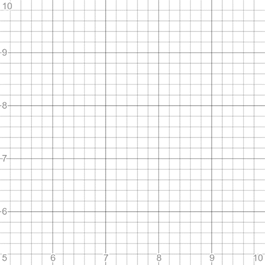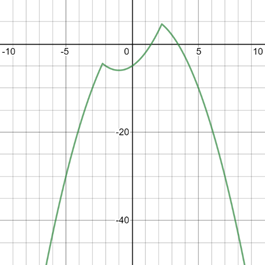# To graph : The equation y = 2 x − | x 2 − 5 | is an appropriate viewing rectangle.### Precalculus: Mathematics for Calcu...

6th Edition
Stewart + 5 others
Publisher: Cengage Learning
ISBN: 9780840068071### Precalculus: Mathematics for Calcu...

6th Edition
Stewart + 5 others
Publisher: Cengage Learning
ISBN: 9780840068071

#### Solutions

Chapter 1.9, Problem 22E
To determine

## To graph: The equation y=2x−|x2−5| is an appropriate viewing rectangle.

Expert Solution

### Explanation of Solution

Given information:

The equation y=2x|x25| .

Graph:

For the graph of equation y=2x|x25| firstly set the suitable values of a,b,c,d for viewing rectangles.

Take values [5,10]×[5,10] then resulting graph is blank because |x25|0 so 2x|x25|2x for all the values of x .Thus, graph lies below the viewing rectangle, so this viewing rectangle is not appropriate.

The graph of the equation y=2x|x25| can be sketched using the traces,

For the value x=0 , the trace of the equation is,

y=0|05|=5

For the value x=1 , the trace of the equation is,

y=2|15|=2

For the plane x=2 , the trace of the equation is,

y=4|41|=1

For the different values of x get different values of y .

The whole graph is lies below from the viewing rectangle.

Take values [-10,10]×[-50,10]The resulting graph is appropriate viewing rectangle.

For enlarge the viewing rectangle change the values of a,b,c,d .

Interpretation:

The graph of an equation in a viewing screen is a viewing rectangle.

The x -values to range from a minimum value of xmin =a to a maximum value of xmax =b

The y -values to range from minimum value of ymin =c to a maximum value of ymax =d

Then, the display portion of the graph lies in the rectangle [a,b]×[c,d]={(x,y)|axb,cyd}

The graph is appropriate in [-10,10]×[-50,10] viewing rectangle

### Have a homework question?

Subscribe to bartleby learn! Ask subject matter experts 30 homework questions each month. Plus, you’ll have access to millions of step-by-step textbook answers!